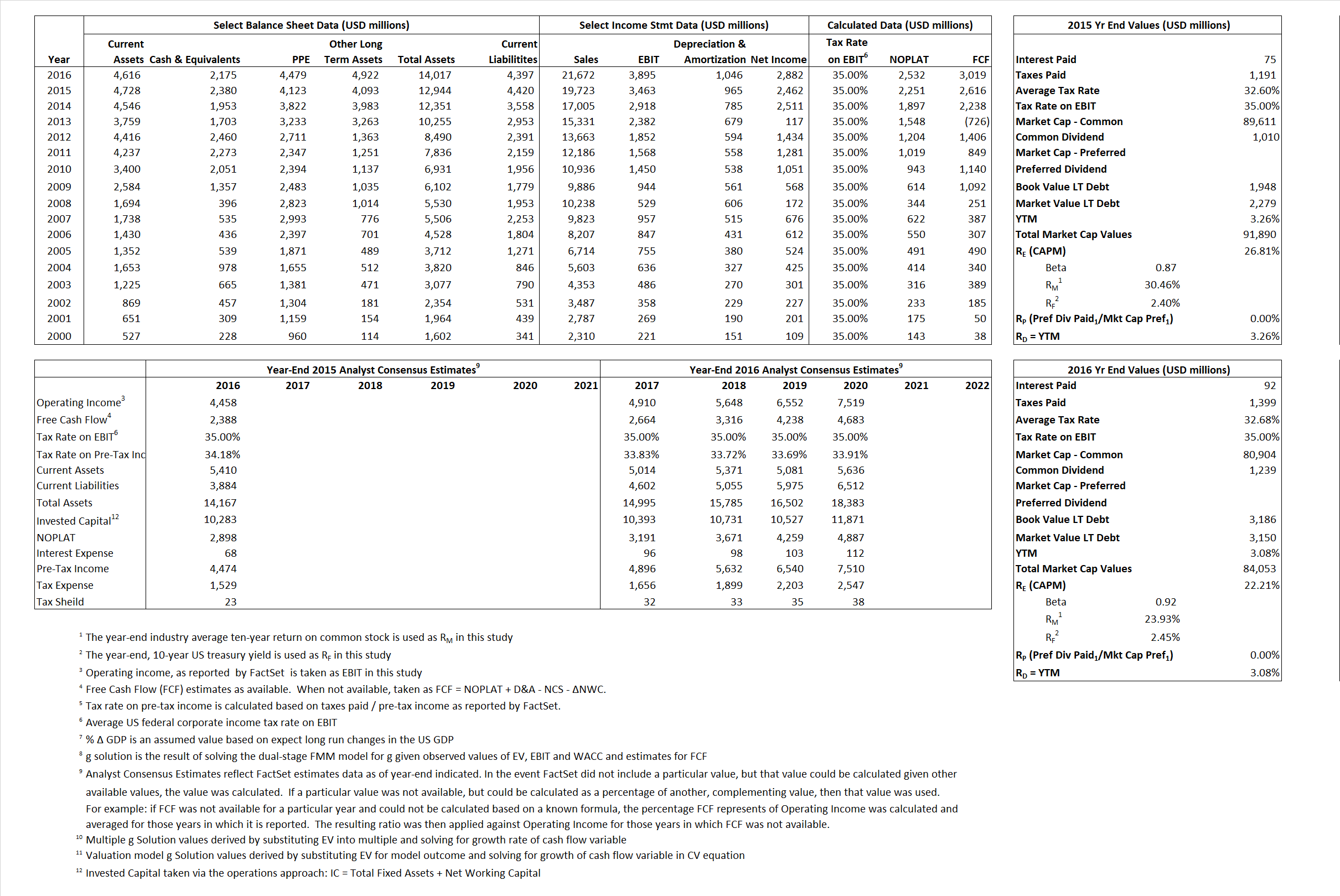# Starbucks Corporation

## Analyst Listing

The following analysts provide coverage for the subject firm as of May 2016:

 Broker Analyst Analyst Email Cowen & Company Andrew M. Charles andrew.charles@cowen.com BMO Capital Markets Andrew Strelzik andrew.strelzik@bmo.com Deutsche Bank Research Brett Levy brett.levy@db.com Oppenheimer Brian Bittner brian.bittner@opco.com RBC Capital Markets David Palmer david.palmer@rbccm.com Credit Suisse Jason West jason.west@credit-suisse.com Nomura Research Mark Kalinowski mark.kalinowski@nomura.com Stifel Nicolaus Mark S. Astrachan msastrachan@stifel.com Evercore ISI Matt McGinley matt.mcginley@evercoreisi.com Guggenheim Securities Matthew J. Difrisco matthew.difrisco@guggenheimpartners.com Wedbush Securities Nick Setyan nick.setyan@wedbush.com BTIG Peter Saleh psaleh@btig.com William Blair Sharon Zackfia szackfia@williamblair.com Stephens Inc Will Slabaugh will.slabaugh@stephens.com

## Primary Input Data## Derived Input Data

### Equational Form

Net Operating Profit Less Adjusted Taxes NOPLAT 2,251  2,532$NOPLAT\, =\, EBIT\, x\, (1 \,-\, Avg \,\,Tax\,\, Rate\,\, on\,\, EBIT)$
Free Cash Flow FCF 2,616  3,019$FCF\,=NOPLAT\,+\,Non-Cash\,Expenses-\Delta NWC\,-\,NCS$
Tax Shield TS 24 30$TS\,=\,Interest\,\,Paid\,\,x\,\, Avg \,\,Tax\,\,Rate\,\, on\,\, Pre-Tax\,\, Income$
Invested Capital IC 8,523 9,620$IC\,=\,Fixed\,\,Operating\,\,Assets\,\,+\,\,Net\,\, Working\,\, Capital$
Return on Invested Capital ROIC  26.41% 26.32%$ROIC\,=\,\frac { NOPLAT }{ IC }$
Net Investment NetInv 695 2,142$NetInv\,=\,{ {IC}_{1}}-{{IC}_{0}}+Depreciation$
Investment Rate IR  30.86% 84.62%$IR\,=\,\frac {NetInv}{NOPLAT}$
Weighted Average Cost of Capital
WACCMarket  26.20% 21.46%$WACC\,=\,\frac { E }{ V } { R }_{ E }\,+\,\frac { P }{ V } { R }_{ P }\,+\,\frac { D }{ V } { R }_{ D }\left( 1- Avg\,\, Tax\,\,Rate\,\,on\,\,Pre-Tax\,\,Income \right)$
WACCBook   8.45%   8.29%
Enterprise value
EVMarket 89,510 81,878$EV\,=\,Market\,\,Cap\,\,Equity\,+\,\,Long\,\,Term\,\,Debt\,-\,Cash$
EVBook   89,384  81,914
Long-Run Growth
g = IR x ROIC
8.15%  22.27% Long-run growth rates of the income variable are used in the Continuing Value portion of the valuation models.
g = %$\Delta$ GDP   2.50%   2.50%
Margin from Operations M  17.56%  17.97%$M\,\,=\,\,\frac{EBIT}{SALES}$
Depreciation/Amortization Rate D  21.79%  21.17%$D\,\,=\,\,\frac{D+A}{EBITDA}$

## Valuation Multiple Outcomes

The outcomes presented in this study are the result of original input data, derived data, and synthesized inputs.

### model g solution

12/31/2015 12/31/2016 12/31/2015 12/31/2016 12/31/2015 12/31/2016

EV/SALES$\frac {EV}{Sales} \,= \,\frac{ROIC\, -\, g}{ROIC\,(WACC\,-\,g)}\,(1\,-\,T)\,(M)$

4.54  3.78  26.18%  20.81%  26.18%  21.18%

EV/EBITDA$\frac {EV}{EBITDA} \,= \,\frac{ROIC\, -\, g}{ROIC\,(WACC\,-\,g)}\,(1\,-\,T)\,(1\,-\,D)$

20.22 16.57 26.18% 20.81% 26.18% 21.18%

EV/NOPLAT$\frac {EV}{NOPLAT} \,= \,\frac{ROIC\, -\, g}{ROIC\,(WACC\,-\,g)}$

39.77 32.34 26.18% 20.81% 26.18% 21.18%

EV/FCFOPS$\frac {EV}{FCF_{OPS}} \,= \,\frac{ROIC\, -\, g}{ROIC\,(WACC\,-\,g)}\,(1\,-\,T)$

34.21 27.12 26.18% 20.81% 26.18% 21.18%

EV/EBIT$\frac {EV}{EBIT} \,= \,\frac{ROIC\, -\, g}{ROIC\,(WACC\,-\,g)}\,(1\,-\,T)$

25.85 21.02 26.18% 20.81% 26.18% 21.18%

EV/IC$\frac {EV}{IC} \,= \,\frac{ROIC\, -\, g}{WACC\,-\,g}$

10.50 8.51 26.18% 20.81% 26.18% 21.18%